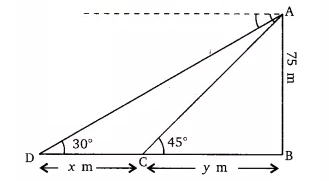# 13. As observed from the top of a 75 m high lighthouse from the sea-level, the angles of depression of two ships are 30° and 45°. If one ship is exactly behind the other on the same side of the lighthouse, find the distance between the two ships.

M manish

Given that,
Height of the lighthouse (AB) is 75 m from the sea level. And the angle of depression of two different ships are  and  respectivelyLet the distance between both the ships be  m.
According to question,

In triangle ,

.............(i)

In triangle ,

.............(ii)

From equation (i) and (ii) we get;

Hence, the distance between the two ships is approx 55 m.

Exams
Articles
Questions